# Torque on a Current Carrying Loop

The fact that a force exists on a wire carrying a current in a magnetic field has an interesting application when we consider a current carrying loop. Consider a rectangular loop carrying a currentin a magnetic field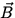as in Fig. 1.5.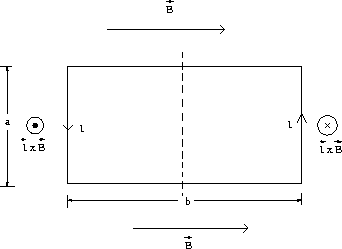We consider the force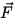= lxof Eq.(1.4) on each of the four sides of the loop. On the top and bottom sections this force vanishes, becauseandare parallel or antiparallel in these cases. The force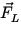on the left section will have a magnitude aIB in the direction indicated, while the force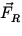on the right section will have the same magnitude aIB but in the opposite direction. These two forces, since they are oppositely directed, do not give rise to a net linear acceleration, but they do tend to rotate the loop around the vertical axis. There will thus be a net torque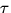on the loop, which is given by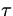= FL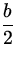+ FR= aIBb = BIA, (5)

where A = ab is the area of the loop.

Although we have considered a rectangular loop, the relation= BIA holds for an arbitrarily shaped loop of area A . The fact that current carrying loops experience a net torque in a magnetic field is the principle behind the electric motor, where the electrical energy involved in establishing a current is converted into the mechanical energy of rotating a shaft.Next: Sources of the Magnetic Up: Magnetism Previous: Magnetic Force on a